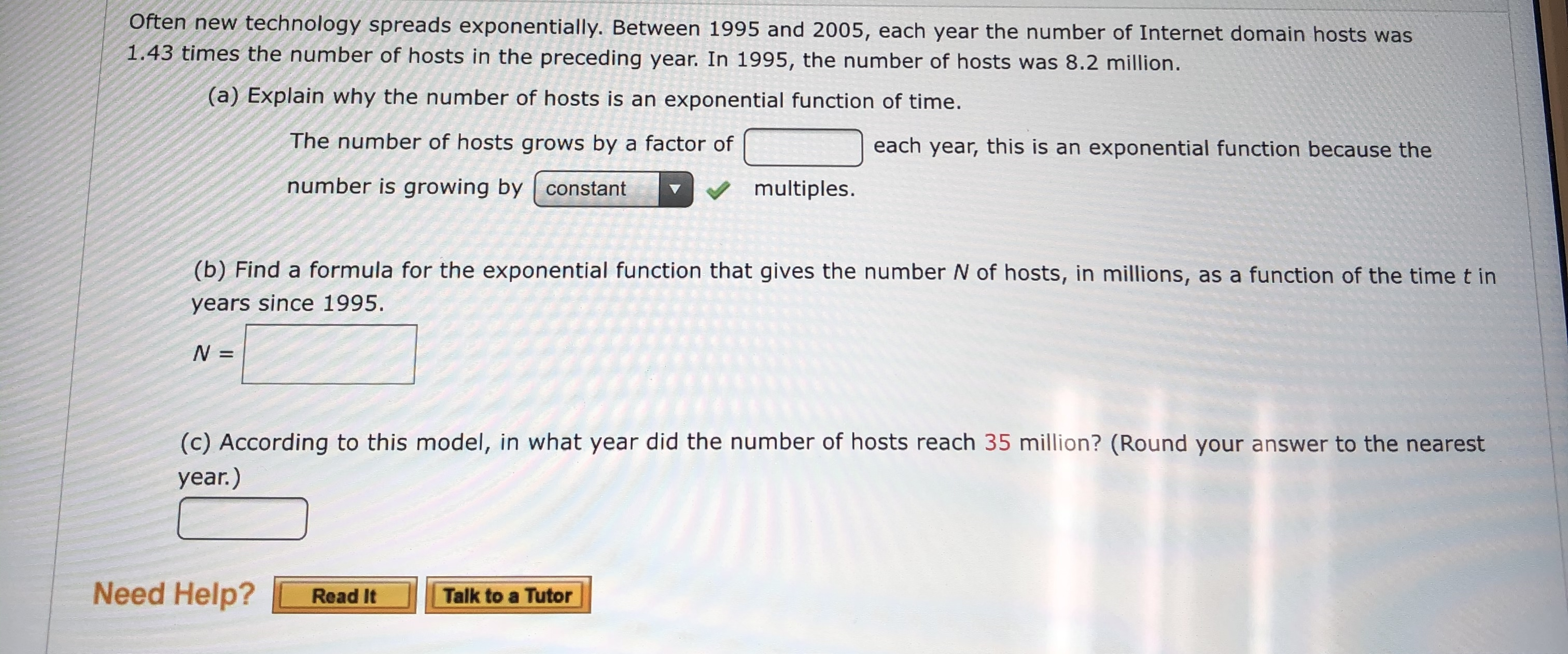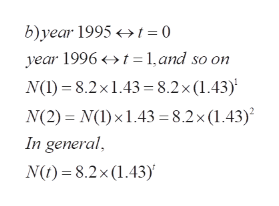# Often new technology spreads exponentially. Between 1995 and 2005, each year the number of Internet domain hosts was1.43 times the number of hosts in the preceding year. In 1995, the number of hosts was 8.2 million.(a) Explain why the number of hosts is an exponential function of time.The number of hosts grows by a factor ofeach year, this is an exponential function because thenumber is growing bymultiples.constant(b) Find a formula for the exponential function that gives the number N of hosts, in millions, as a function of the timet inyears since 1995.N =(c) According to this model, in what year did the number of hosts reach 35 million? (Round your answer to the nearestyear.)Need Help?Talk to a TutorRead It

Question

What are the blanks?help_outlineImage TranscriptioncloseOften new technology spreads exponentially. Between 1995 and 2005, each year the number of Internet domain hosts was 1.43 times the number of hosts in the preceding year. In 1995, the number of hosts was 8.2 million. (a) Explain why the number of hosts is an exponential function of time. The number of hosts grows by a factor of each year, this is an exponential function because the number is growing by multiples. constant (b) Find a formula for the exponential function that gives the number N of hosts, in millions, as a function of the timet in years since 1995. N = (c) According to this model, in what year did the number of hosts reach 35 million? (Round your answer to the nearest year.) Need Help? Talk to a Tutor Read It fullscreen
check_circle

Step 1

The problem concerns aspects of an exponential growth model

Step 2

a) blank = constant  (basically geometric series model)

Step 3

b) N(t) expressed as a function of ti...help_outlineImage Transcriptioncloseb)year 1995>t =0 year 1996t 1,and so on N(I)8.2x1.43 =8.2 x (1.43) N(2) N(I)x1.43 = 8.2x (1.43) In general N)8.2x (1.43)' fullscreen

### Want to see the full answer?

See Solution

#### Want to see this answer and more?

Solutions are written by subject experts who are available 24/7. Questions are typically answered within 1 hour.*

See Solution
*Response times may vary by subject and question.
Tagged in

### Applications of Mathematics